## ML Aggarwal Class 8 Solutions for ICSE Maths Chapter 14 Constructions of Quadrilaterals Check Your Progress

Question 1.
Construct a quadrilateral ABCD such that AB = 4·5 cm, BC = 4 cm, CD = 3·9 cm, AD = 3·2 cm and ∠B = 60°.
Solution: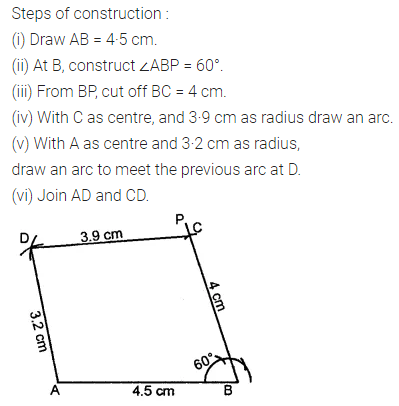Question 2.
Construct a quadrilateral ABCD such that AB = 5 cm, BC = 4·2 cm, AD = 3·5 cm, ∠A = 90°, ∠B = 60°.
Solution: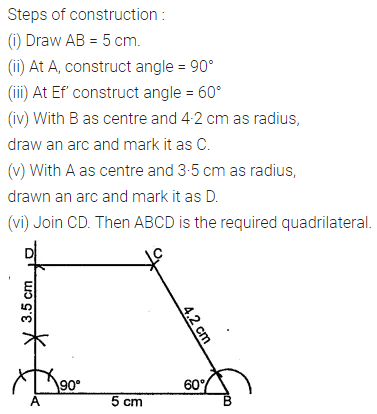Question 3.
Construct a quadrilateral ABCD in which AB 3·5 cm, BC = 5 cm, CD = 5·6 cm, DA = 4 cm and BD = 5·4 cm
Solution: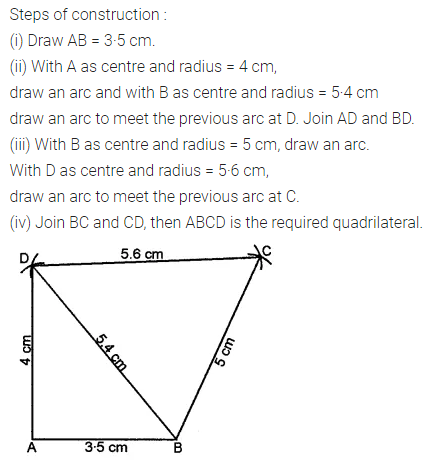Question 4.
Construct a quadrilateral PQRS in which PQ = 3 cm, QR = 2·5 cm, PS = 3·5 cm, PR = 4 cm and QS = 5 cm.
Solution: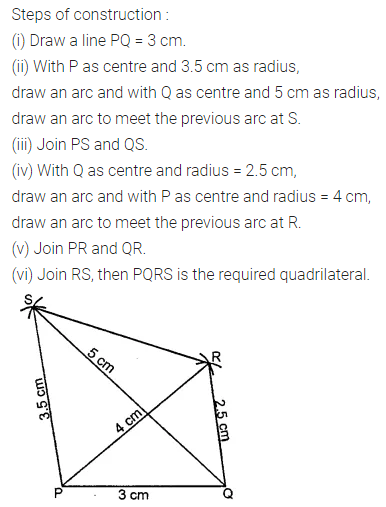Question 5.
Construct a quadrilateral ABCD given that BC = 6 cm, CD = 4 cm, ∠B = 45°, ∠C = 90° and ∠D = 120°.
Solution: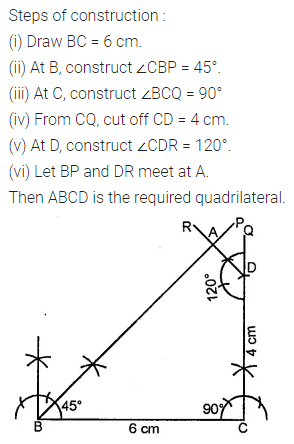Question 6.
Construct a parallelogram ABCD in which AB = 5.2 cm, AC = 6.5 cm and BD = 7.8 cm.
Solution: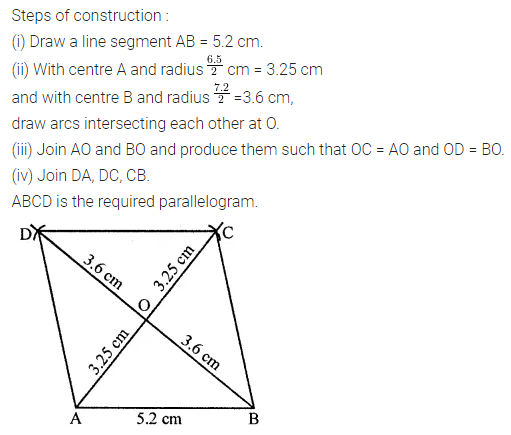Question 7.
Construct a parallelogram ABCD in which diagonal AC = 4 cm, diagonal BD = 6 cm and angle between diagonals is 75°.
Solution: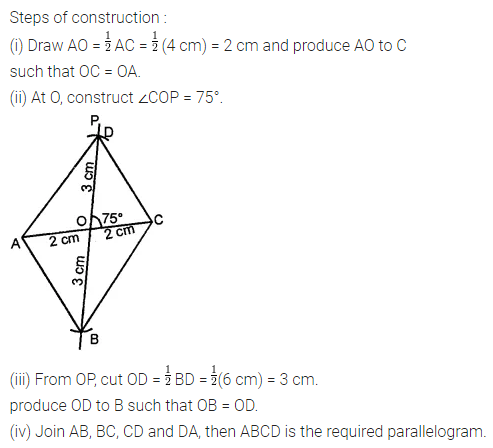Question 8.
Construct a rectangle PQRS such that PQ = 3·5 cm and ∠RPS = 75°.
Solution: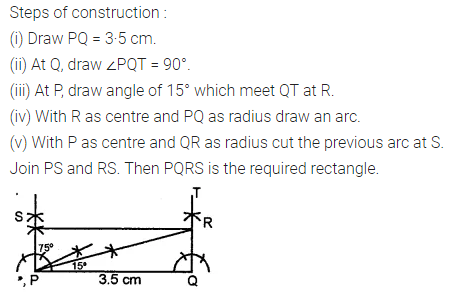Question 9.
Construct a rectangle whose one diagonal is 6-8 cm and an angle between two diagonals is 105°.
Solution: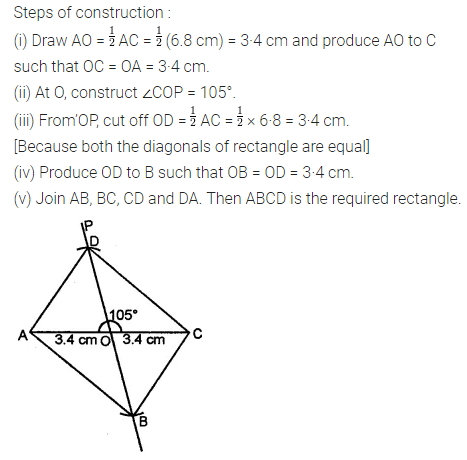Question 10.
Construct a rhombus BEST such that BE = 4.5 cm, ET = 6 cm.
Solution: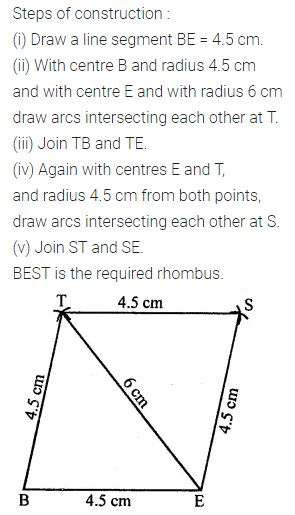Question 11.
Construct a rhombus whose diagonals are 7 cm and 5·3 cm.
Solution: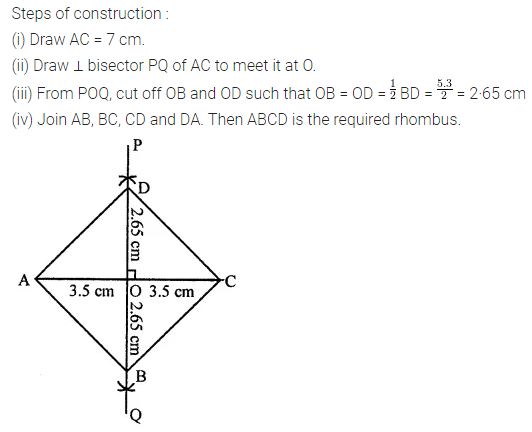Question 12.
Construct a square whose one diagonal is 5·8 cm.
Solution: